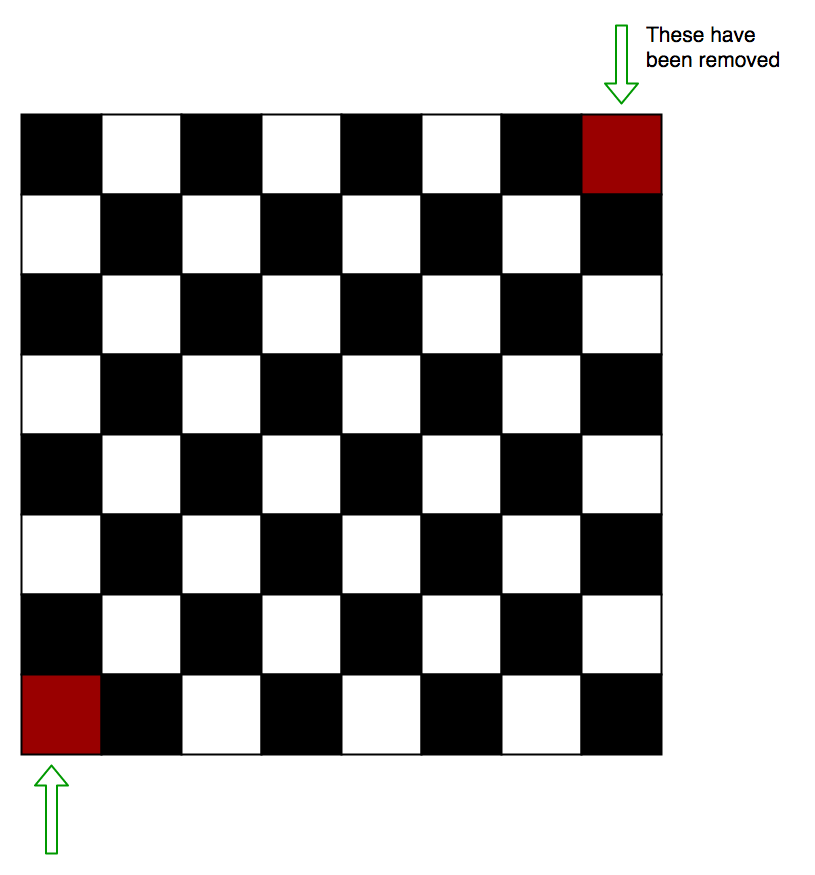# Puzzle 25 | (Chessboard and dominos)

There is an 8 by 8 chessboard in which two diagonally opposite corners have been cut off.You are given 31 dominos, and a single domino can cover exactly two squares. Can you use the 31 dominos to cover the entire board?

No

Explanation:
At first it seems that there were 8*8 = 64 squares
then 2 have been cut off so Squares remaining= 64-2 = 62
And there are 31 dominos, so they will cover the remaining chessboard coz = 31*2 = 62

But this is not the answer:

Lets visualize it:Each domino we set on the chessboard will always take 1 Black and 1 White square.Therefore, 31 dominos will take 31 white square and 31 black squares exactly. On this chessbord however, we must have 32 black and 30 white squares. Hence it is not possible to do so.